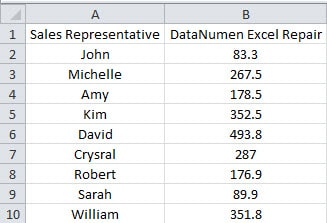## The Difference between Excel Built-in ROUND Function and VBA ROUND Function

You will certainly have used the ROUND function in your Excel. If you also use VBA to finish some tasks, you may also have used the ROUND function in codes. However, the built-in ROUND functions in worksheet and in VBA codes work differently. Below we will show you how they work differently.

## Built-in ROUND Function

In this worksheet, you can see the sales volume of different sales representatives. The numbers are decimal numbers in the worksheet.To calculate more conveniently, you need to round those numbers. And certainly you can use the ROUND function in Excel.

1. Click a blank cell in the worksheet that you need to input the numbers. Here we will click cell C2.
2. And then input the formula into the cell:

=ROUND(B2,0)

Here we will round numbers into integers.

1. After that, double click the fill handle of the cell C2 and fill this formula for the whole column. The results are shown in the image below.## VBA ROUND Function

Sometimes using VBA can save you a lot of time and energy. Here you can use VBA to round numbers.

1. Press the shortcut keys “Alt +F11” on the keyboard to open the Visual Basic editor.
2. And then insert a new module into the editor.3. Now you will use VBA macros. Here we will use this example:
```Sub VBARoundFunction()
Dim nRowIndex As Integer

'  Round the numbers in the target range
For nRowIndex = 2 To Application.WorksheetFunction.CountA(Range("B:B"))
Range("D" & nRowIndex) = Round(Range("B" & nRowIndex))
Next nRowIndex
End Sub```
1. After that, run this macro. According to the macro, the result will be produced in column D.

If you see clearly of the results, you may find some differences. We will analyze the difference in the next part.

## The Different Results

The image below show the differences of the built-in ROUND function and the VBA ROUND function.For the number in cell B5, the result of built-in function is “353”, while the result of the VBA function is “352”. In VBA, the ROUND function will always keep the result as an even number when the first number in the discarded numbers is 5. As for other numbers, it will work the same as the built-in function. Therefore, the result of cell B4 is 172 and the result of cell B5 is 352. They are both even numbers.

Now you have known the reason and the result of the two different ROUND functions. The next time when you need to use VBA macros, you need to pay special attention. In order to avoid the errors, you can use the built-in function in VBA codes.

## Excel Application will also Corrupt

If you have frequently used Excel in your work, you must know how powerful it is.

However, the application of Excel will still corrupt due to many reasons. At this moment, you can use our excellent tool to repair corrupted xlsx file. All the errors can be easily solved by this tool.

## Author Introduction:

Anna Ma is a data recovery expert in DataNumen, Inc., which is the world leader in data recovery technologies, including repair Word doc document problem and outlook repair software products. For more information visit www.datanumen.com